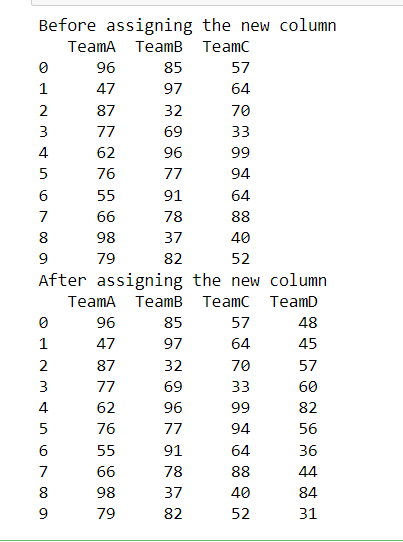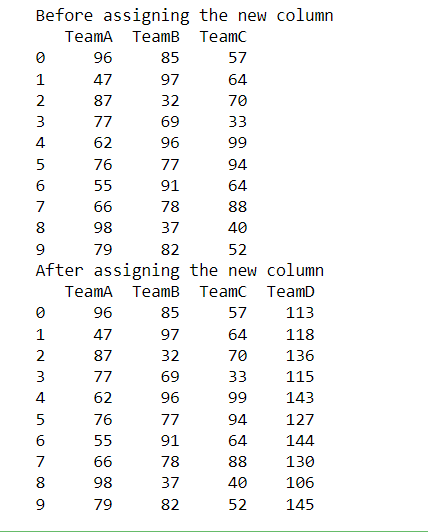# Adding New Variable to Pandas DataFrame

• Last Updated : 13 Jun, 2022

In this article let’s learn how to add a new variable to pandas DataFrame using the assign() function and square brackets.

Pandas is a Python package that offers various data structures and operations for manipulating numerical data and time series. It is mainly popular for importing and analyzing data much easier. Whereas Pandas DataFrame is a potentially heterogeneous two-dimensional size-mutable tabular data structure with labeled axes (rows and columns). A data frame is a two-dimensional data structure in which data is organized in rows and columns in a tabular format. The data, rows, and columns are the three main components of a Pandas DataFrame. here we will see two different methods for adding new variables to our pandas Dataframe.

### Method 1: Using pandas.DataFrame.assign() method

This method is used to create new columns for a DataFrame. It Returns a new object containing all original columns as well as new ones. If there are  Existing columns, they will be overwritten if they are re-assigned.

Syntax: DataFrame.assign(**kwargs)

• **kwargsdict of {str: callable or Series} : Keywords are used to name the columns. If the values are callable, they are computed and assigned to the new columns on the DataFrame. The callable must not modify the input DataFrame . If the values are not callable (for example, if they are a Series, scalar, or array), they are easily assigned.

Returns: A new DataFrame is returned with the new columns as well as all the existing columns.

Example

In this example, we import the NumPy and the panda’s packages, we set the seed so that the same random data gets generated each time. A dataset with 10 team scores ranging from 30 to 100 is generated for three teams. The assign() method is used to create another column in the Dataframe, we provide a keyword name which will be the name of the column we’ll assign data to it. After assigning data, a new Dataframe gets created with a new column in addition to the existing columns.

## Python3

 `# import packages` `import` `numpy as np` `import` `pandas as pd`   `# setting a seed` `np.random.seed(``123``)` `# creating a dataframe` `df ``=` `pd.DataFrame({``'TeamA'``: np.random.randint(``30``, ``100``, ``10``),` `                   ``'TeamB'``: np.random.randint(``30``, ``100``, ``10``),` `                   ``'TeamC'``: np.random.randint(``30``, ``100``, ``10``)})` `print``(``'Before assigning the new column'``)`   `print``(df)` `# using assign() method to add a new column` `scores ``=` `np.random.randint(``30``, ``100``, ``10``)`   `df2 ``=` `df.assign(TeamD``=``scores)`   `print``(``'After assigning the new column'``)`   `print``(df2)`

Output:### Method 2: Using [] to add a new column

In this example, instead of using the assign() method, we use square brackets ([]) to create a new variable or column for an existing Dataframe. The syntax goes like this:

```dataframe_name['column_name'] = data
column_name is the name of the new column to be added in our dataframe.```

Example

we get the same output as when we used the assign() method. A new column called TeamD is created in this example, which shows the scores of people in TeamD. Random data is created and assigned to the Dataframe to the new column.

## Python3

 `# import packages` `import` `numpy as np` `import` `pandas as pd`   `# setting a seed` `np.random.seed(``123``)` `# creating a dataframe` `df ``=` `pd.DataFrame({``'TeamA'``: np.random.randint(``30``, ``100``, ``10``),` `                   ``'TeamB'``: np.random.randint(``30``, ``100``, ``10``),` `                   ``'TeamC'``: np.random.randint(``30``, ``100``, ``10``)})` `print``(``'Before assigning the new column'``)`   `print``(df)` `# using [] to add a new column` `scores ``=` `np.random.randint(``100``, ``150``, ``10``)`   `df[``'TeamD'``] ``=` `scores`   `print``(``'After assigning the new column'``)`   `print``(df)`

Output:My Personal Notes arrow_drop_up
Recommended Articles
Page :